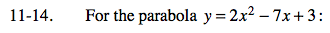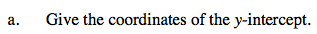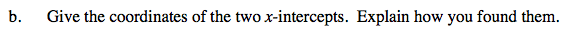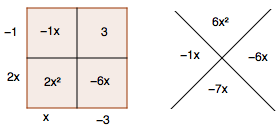### Home > AC > Chapter 11 > Lesson 11.1.1 > Problem11-14

11-14.At the y-intercept, x = 0.

y-intercept: (0, 3)At the x-intercept, y = 0.

Substitute 0 for y and factor the equation.

(2x − 1)(x − 3) = 0Use the Zero Product Property to solve for x.

x-intercepts: (0.5, 0) and (0, 3)